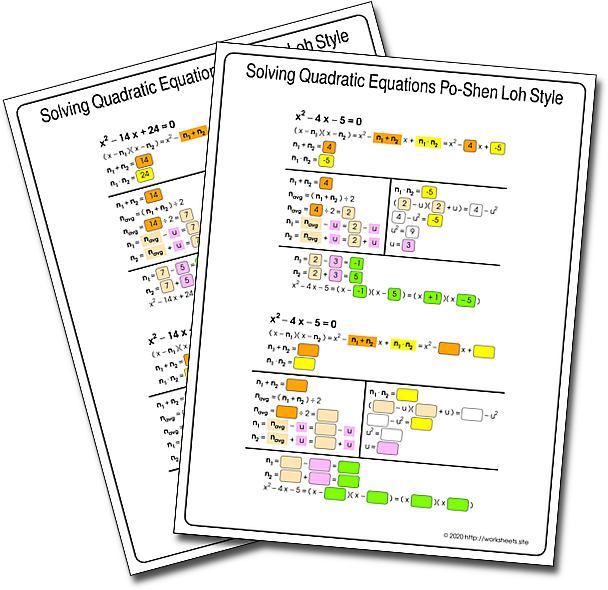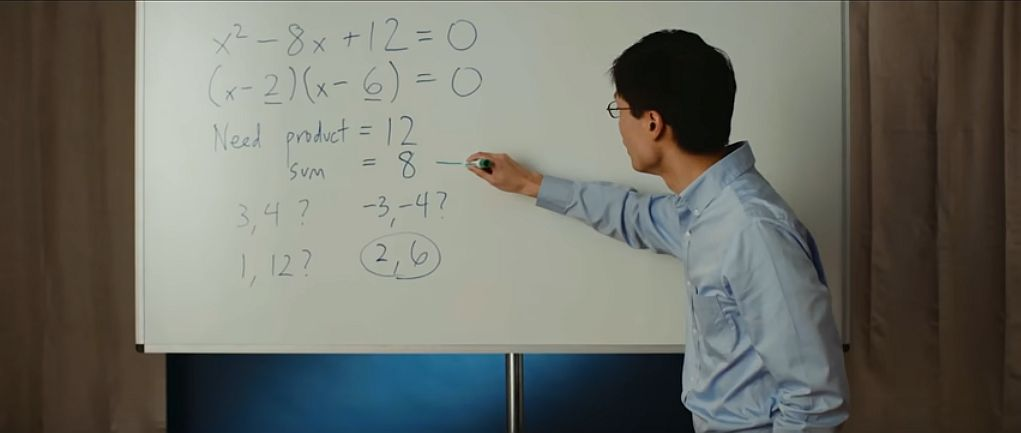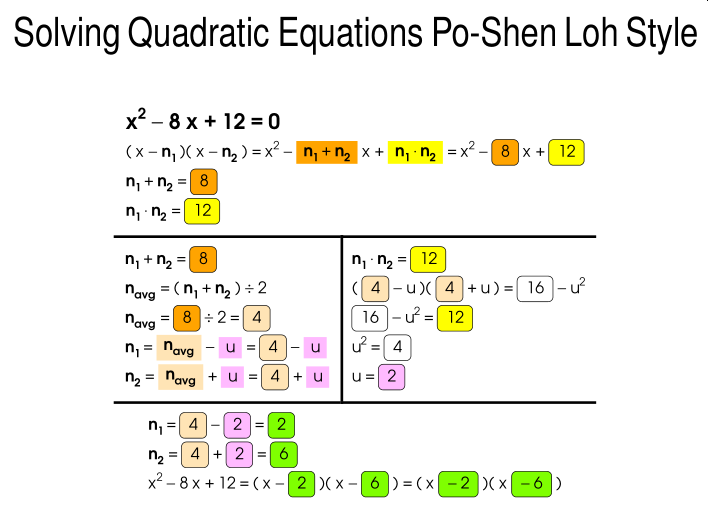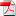# Worksheets for solving Quadratic Equations Po-Shen Loh Style

A Different Way to Solve Quadratic Equations without using the Quadratic Formula. Worksheets for solving Quadratic Equations Po-Shen Loh Style. Factoring Trinomials without Guessing. New way of factoring Trinomials. Trick Makes Quadratic Equations Easier. Easier way to find the roots of a Quadratic Equation.Math teacher Po-Shen Loh had been thinking about how to explain school math concepts in a more thoughtful and interesting manner. While brainstorming different ways to think about the quadratic formula, he came up with a simple way to solve quadratic equations that the world had never seen before. He was very surprised, as this method was easier to understand than what is typically written in textbooks through the blind use of the quadratic formula. Adding this technique as a standard method would directly improve the learning experience for anyone trying to understand this topic, which is part of the regular mathematical curriculum everywhere in the world.“Math is not about memorizing formulas without meaning, but rather about learning how to reason logically through precise statements,” Dr. Loh said.

While continuing to investigate mathematical history around quadratic equations. He found that the individual steps of this method had been separately discovered by ancient mathematicians, and some deep digging unearthed modern teachers who were on the same track. He published his investigations in an article formally called "A Simple Proof of the Quadratic Formula". And he prepared a detailed explanation of the new method at his website and prepared several videos on the topic. There, he goes through each step and explains the reasons why it works and why, in his view, it is better than the blind use of the formula.

His insight was featured in several news articles including the New York Times and the Daily Mail with headlines like "Mathematician discovers a simpler way to solve the quadratic equation that could fundamentally change the way students are taught math" and "This Professor´s Amazing Trick Makes Quadratic Equations Easier". It was even explained by the very popular youtube channel 3Blue1Brown as an aplication of the fact the a product of two numbers can be thought of as the difference of two squares.

We ourselves have prepared these worksheetsto complement a lesson plan around Loh's style of solving quadratic equations, and incidentally, of factoring trinomials. Use the worksheets.site password to open the PDF file. The PDF file has 11 worksheets to print and practice the method, it has 2 pages of examples to get kids going.